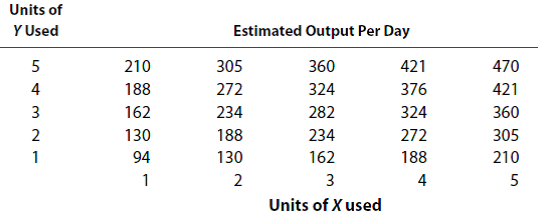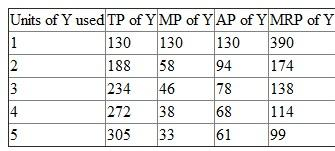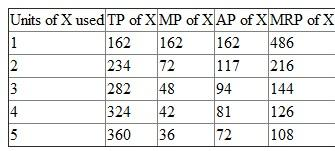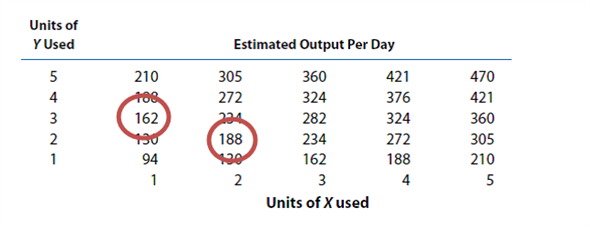## Quiz 7 : Production Analysis and Compensation PolicyLooking for Economics Homework Help?# Quiz 7 : Production Analysis and Compensation Policy

(a) The inputs exhibit the characteristic of a decreasing marginal rate of technical substitution. It can be said because in the case of decreasing marginal rate of technical Substitution the slope of the production isoquants diminishes as one input is increasingly substituted for another. For example, suppose 2 units of X is used and 2 units of Y, then the output produced is 188 units. Now, substituting 1 unit of X for Y, total inputs used will be 1 unit of X and 3 units of Y. Here, the output produced will be equal to 162. Therefore, when one input is substituted for another, production declines and thus, there are decreasing marginal rates of substitution.(b) The below table shows when X is fixed at 2 units is formulated on the basis of thatMarginal product is calculated by deducting the next unit of total product from the first unit of total product. Average product is calculated by dividing the total product by units of Y. MRP is calculated by multiplying MP with \$3. The below table shows when Y is fixed at 3 units is formulated on the basis of that:Marginal product is calculated by deducting the next unit of total product from the first unit of total product. Average product is calculated by dividing the total product by units of Y. MRP is calculated by multiplying MP with \$3. (c) If X is fixed at 2 units and the output sells for \$3 and the cost of Y is \$120 per day then only till 3 units of Y should be employed as MRP at 4 th unit is 114 which is less than the cost of \$120. (d) When 1 unit of X and 3 units of Y is employed then total product is only 162. So, the company can change its order as by using 2 units of both inputs it can increase its total product to 188.In other words, using the given combination of 1 unit of X and 3 units of Y, the firm can produce 162 units. But if the firm gives up one unit of input Y, it can get one unit of input X, and therefore, using 2 units X and 2 units of Y, the firm can produce 188 units of goods. Thus, a change in the present input combination is recommended. (e) The nature of the returns to scale for this production system is the optimal combination requires that X=Y is constant returns to scale. This is true because a given increase in both inputs causes an increase in output in the same proportion.

Employment of least-cost input combinations constitutes a necessary but insufficient condition for profit maximization. Profit maximization requires that a firm employ optimal input proportions and produce the most advantageous quantity of output. Profits are maximized when inputs are employed so that price equals marginal revenue product for ech input. The difference between cost minimization and profit maximization is simple. Cost minimization requires efficient resource use as reflected by optimal input proportions. profit maximization requires efficient resource use and production of an optimal level of output as made possible by the most favorable employment of all inputs.

(A) The answer is True When there is E Q 1, the percentage change in output is less than a given percentage change in all inputs. Thus, decreasing returns to scale and increasing average costs are indicated. (B) The answer is True If the marginal product of capital falls as capital usage grows, the returns to capital are decreasing. (C) The answer is False. L-shaped production isoquants describe the production system in which inputs are perfect complementary. (D) The answer is False Marginal revenue product is the amount of revenue generated by employing the last input unit. Marginal revenue product does not measure the increase in profit earned through expansion. (E) The answer is False Marginal revenue product is not affected by the given percentage increase in the marginal productivity of all inputs.